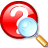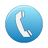# Mathematics & Statistics

## Definition

Mathematics
The study of numbers, shapes, and other entities by logical means. It is divided into pure mathematics and applied mathematics, although the division is not a sharp one and the two branches are interdependent. Applied mathematics is the use of mathematics in studying natural phenomena. It includes such topics as statistics, probability, mechanics, relativity, and quantum mechanics. Pure mathematics is the study of relationships between abstract entities according to certain rules. It has various branches, including arithmetic, algebra, geometry, trigonometry, calculus, and topology.

mathematics. (2008). In D. Nelson (Ed.), The Penguin dictionary of mathematics (4th ed.). London, UK: Penguin. Retrieved from http://proxy.devry.edu/login?url=http://search.credoreference.com/content/entry/penguinmath/mathematics/0?institutionId=2985

Statistics
Branch of mathematics concerned with the collection and interpretation of data.

statistics. (2016). In Helicon (Ed.), The Hutchinson unabridged encyclopedia with atlas and weather guide. Abington, UK: Helicon. Retrieved from http://proxy.devry.edu/login?url=http://search.credoreference.com/content/topic/statistics?institutionId=2985

## Keywords

algebra, business mathematics, calculus, elementary statistics, equations, math, mathematics, statistics, trigonometry

## Ask A LibrarianKnowledge Base search a series of commonly asked questions by topic keyword or course numberTelephone a librarian at 800-293-3044